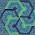## Thursday, February 20, 2014

### Quilty Geometry Lesson

Math. It's everywhere.

I have always loved math. As a quilter, math seems to come up all the time.

This past week, my niece, Karen, had a problem. The pattern she was working on had a mistake. Her half-square triangles were cut according to the pattern, but they were too big. Since she's still new at this quilty thing, we texted back and forth and figured out the problem, but I thought you might be interested in how I explained the math to make half-square triangles.

Most of us quilty-types learned that you can easily make a half-square triangle by cutting a square, then cutting that square in half along the diagonal. You get two with every cut!  Usually, you then sew that half-square triangle to another one to make what I call a half-square triangle unit--two half-square triangles sewn together along the long bias line of the diagonal cut.

Things start to get tricky when you have to figure out the size of the square to cut.

Early in my quilty life, I learned that you take the finished size (the size without seam allowances) of the half-square triangle unit and add 7/8" and that's the size to make the square. But do you really, REALLY understand where that 7/8" came from?

Hang on to your seats, this might be a bumpy flight--it all boils down to . . . the Pythagorean Theorem. Yes, we're taking a trip in the way-back machine and making a visit to high school geometry class!

Don't be concerned if this math stuff is like a foreign language. In the Wizard of Oz movie classic, even the Scarecrow didn't get it right. Once he got his brain from the wizard, he proceeded to botch the Pythagorean Theorem, not once, but twice. He talks about an isosceles triangle, when it's supposed to be a right triangle. And he talks about square roots, but it's squares (multiplying a number by itself) we need. So, you aren't alone if this stuff confuses you! You have good company!

So, let's see if we can make this make some sense--in quilty terms.

First, let's make a couple of half-square triangles. I want them to finish to 5" square, so, following the generally accepted rule, I add 7/8" and cut a couple of 5-7/8" squares.

These two squares cut on the diagonal will yield two half-square triangle units that are 5" square. Notice the tip of the triangle, 5-7/8".

Some may prefer to leave the square uncut. Still, you start with 5-7/8" squares.

See?

Sew 1/4" on both sides of the line, then cut on the line.

You can see that my seam allowance is 1/4" . . . We'll refer to this down below. So, don't forget this spot!

The half-square triangle unit, before it's pressed. . .

I like to trim off the points (circled) before I press the seam to one side. Notice the size of the triangle after trimming the points, is 5-1/2"--that's the unfinished size of the triangle unit.

Here's a close up of the trimmed point--I like to trim them to remove bulk, and because I'm a tidy-butt. Goes with the fondness for math.

Once the seam has been pressed--I prefer to press to one side--the unit is 5-1/2" square (red arrow). So my planned 5" finished size is right on track.

Hold on. Let's rewind the tape a couple of steps. Before we trim the points off, let's take a closer look at that corner.

If I draw a tiny green line perpendicular to the seam, I'm creating a itty-bitty right triangle, a triangle that has one 90˚ angle. That 90˚ angle is between the sides labeled 'a' and 'b'. 'C' is the hypotenuse of the triangle, it's the longer side, opposite the right angle in our itty bitty triangle.

Enter Pythagoras, the Ancient Greek mathematician. The Pythagorean Theorem states that the sum of the squares of two sides of a right triangle is equal to the square of the hypotenuse.

Or: a-squared + b-squared = c-squared

Going back to that picture I told you to remember, the one where I'm measuring the seam allowance, we know that a= 1/4" or .25"

We also know that 'b' is the same as 'a'--maybe, 'know' isn't the right word, for now, take my word for it.

SO: .25(squared) + .25 (squared) = c (squared)

When you multiply .25 by itself, you get .063, Do that again and add the two numbers together:

.063 +.063 = .126

SO: .126 = c(squared)

(Almost there!) Now take the square root of both sides of the equation (It's a simple button on most calculators) and the result is 'c'

OR: .355"

That's how long 'c' is in the photo above.

So what? If you state 3/8" as a decimal (divide 3 into 8 with a few more clicks on the calculator) it's .375

Add back 1/2" or .5 for the seam allowance to the number we calculated above:

.355 +.5 = .855

(We already know that .375 + .5 = .875 in other 'words,' 3/8" + 1/2" = 7/8")

TA DA. That's where the 7/8" (.875")comes from! Well, maybe not exactly, but it's dern close!

(Did I lose you?)

Of course, the right isosceles triangle (also known as a half-square triangle) isn't the only triangle in the quilty world, but that's enough math for today. Don't you agree?

I don't know about you, but I have some sewing to do!

Happy Stitching!

1.2.